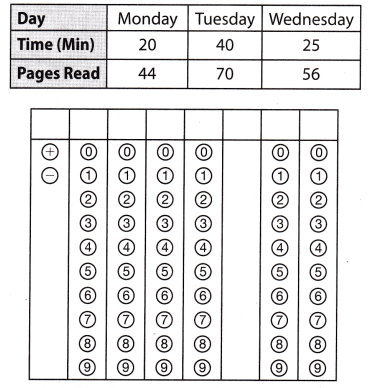Refer to our Texas Go Math Grade 8 Answer Key Pdf to score good marks in the exams. Test yourself by practicing the problems from Texas Go Math Grade 8 Module 3 Quiz Answer Key.

3.1 Representing Proportional Relationships

Question 1.
Find the constant of proportionality for the table of values.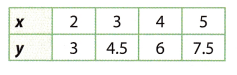$$\frac{3}{2}$$ = 1.5
$$\frac{4.5}{3}$$ = $$\frac{3}{2}$$ = 1.5
$$\frac{6}{4}$$ = $$\frac{3}{2}$$ = 1.5
$$\frac{7.5}{5}$$ = $$\frac{3}{2}$$ = 1.5
Therefore the constant of proportionality is 1.5
The constant of proportionality is 1.5.

Phil is riding his bike. He rides 25 miles in 2 hours, 37.5 miles in 3 hours, and 50 miles in 4 hours. Find the constant of proportionality and write an equation to describe the situation.
Let’s first find the tabLe of the given situation: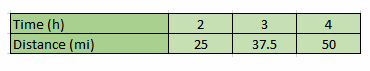The constant of proportionality is 12.5
An equation to describe the situation is y = 12.5x where y is the distance and æ the time.
$$\frac{25}{2}$$ = 12.5
$$\frac{37.5}{3}$$ = $$\frac{25}{2}$$ = 12.5
$$\frac{50}{4}$$ = $$\frac{25}{2}$$ = 12.5
The constant of proportionality is 12.5.
An equation to describe the situation is y = 12.5x where y is the distance and x the time

Constant of proportionality is 12.5, and the equation y = 12.5x

3.2 Rate of Change and Slope

Find the slope of each tine.

Question 3.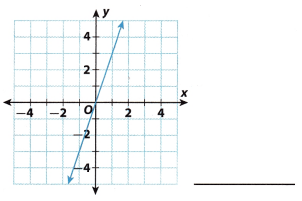We choose two points on the line and we find the change in y-values and the change in x-values as we move from
one point to the other.
rise = +3, since we move up
run = +1, since we move right
Therefore,
slope = $$\frac{+3}{+1}$$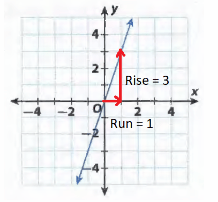slope = 3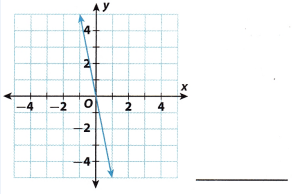Using the graph find 2 points that are on the given line, for example: (0, 0) and (1, -5). Now find the slope
Slope = $$\frac{-5-0}{1-0}$$ = -5

The slope is —5.

3.3 Interpreting the Unit Rate as Slope

Question 5.
What is the slope of the data in the table?The formula for the slope m= y2 – y1/x2 x1.
Here
x1 = 13.5
x2 = 18
y1 = 3
y2 = 4
m = 4 – 3/18 -13.5
m = 1/ 4.5.
m = 0.22.
And
x1 = 18
x2 = 22.5
y1 = 4
y2 = 5
m = 5 – 4/22.5 – 18
m = 1/ 4.5.
m = 0.22.
And
x1 = 22.5
x2 = 27
y1 = 5
y2 = 6
m = 6 – 5/27 -22.5
m = 1/ 4.5.
m = 0.22.
The slope of the data is 0.22

3.4 Direct Variation

Question 6.
A wheelchair ramp rises 2.25 feet for every 25 feet of horizontal distance it covers. What is the slope of the ramp? ________
Given that,
Wheelchair ramp rises = 2.25 feet per every 25 feet of horizontal distance.
Slope of the ramp = m = 2.25/25 = 0.09
The slope of the ramp = 0.09

Essential Question

What is the relationship among direct variation, lines, rates of change, and slope?
The relationship among direct variation, lines, rates of change, and the slope is the variables of the x-axis and the y-axis is the proportional relational ship that has the slope it means the direct variation is determined by calculating the rate of change in the line.

Texas Go Math Grade 8 Module 3 Mixed Review Texas Test Prep Answer Key

Selected Response

Question 1.
Lupe’s heart beats 288 times in 4 minutes. Which equation represents the number of times her heart beats per minute?
(A) y = $$\frac{1}{288}$$x
(B) y = $$\frac{1}{72}$$x
(C) y = 72x
(D) y = 288x
Given that,
Lupe’s heartbeat = 288 times per 4 minutes.
Let x be the number of minutes.
Heartbeat per minute equal to = 288x/4
= 72x
Option C is the correct answer.

Question 2.
Prasert earns $9 an hour. Which table represents this proportional relationship?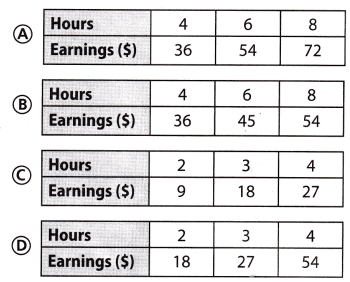Answer: (A)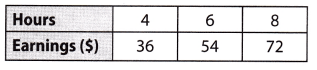Explanation: y = 9x Relationship representing earning at$9 per hr. where x is the number of hour

y = 9(2) = 18 When x = 2, 3, 4, 6, 8
y = 9(3) = 27
y = 9(4) = 36
y = 9(6) = 54
y = 9(8) = 72
Table $A$ represents the situation

A factory produces widgets at a constant rate. After 4 hours, 3,120 widgets have been produced. At what rate are the widgets being produced?
(A) 630 widgets per hour
(B) 708 widgets per hour
(C) 780 widgets per hour
(D) 1,365 widgets per hour
(C) 780 widgets per hour

Explanation:
Let’s denote with x the change ¡n time (hours) and with y the change in the number of widgets produced. We
know that x = 4 and y = 3120. To find the rate of change at which the widgets are being produced, we have:
rate = $$\frac{y}{x}$$
= $$\frac{3120}{4}$$
= 780
In conclusion, 780 widgets are produced every hour.

Question 4.
A full lake begins dropping at a constant rate. After 4 weeks it has dropped 3 feet. What is the unit rate of change in the lake’s level compared to its full level?
(A) 0.75 feet per week
(B) 1.33 feet perweek
(C) -0.75 feet per week
(D) -1.33 feet per week
(A) 0.75 feet per week

Explanation:
$$\frac{3}{4}$$ = 0.75 Find the unit rate byThe lake leaks at $0.75$ feet per week

Question 5.
What is the slope of the line below?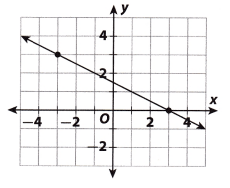(A) -2
(B) – $$\frac{1}{2}$$
(C) $$\frac{1}{2}$$
(D) 2
(B) – $$\frac{1}{2}$$

Explanation:
Using the graph find two points on the line. For example: (3, 0) and (-3, 3). Now using those two points calculate
the slope:
Slope = $$\frac{0-3}{3-(-3)}$$ = $$\frac{-3}{6}$$ = –$$\frac{1}{2}$$

Jim earns $41.25 in 5 hours. Susan earns$30.00 in 4 hours. Pierre’s hourly rate is less than Jim’s, but more than Susan’s. What is his hourly rate?
(A) $6.50 (B)$7.75
(C) $7.35 (D)$8.25
(B) $7.75 Explanation: $$\frac{41.25}{5}$$ = 8.25 Unit rate is given by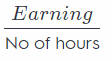Jim’s unit rate is$\$8.25$per hr\dfrac{30}{4} = 7.5Susan’s unit
rate is$\$7.5$per hr Pierre’s hourly rate =$7.75 per hour Pierre’s hourly rate is less than Jim’s but more than Susan’s
7.5 < x < 8.25

Gridded Response

Question 7.
Joelle kept track of her daily reading in the table shown below. What is her fastest daily reading rate in pages per minute?